# Quiz 5: Integer Programming

Business

Person KT needs to prepare an investment plan. In this plan she needs to invest in five funds each from different investment category. Develop an investment plan for Person KT using linear programming model in excel sheet as shown below: Formulate an excel sheet using given formulas in excel spreadsheet: Figure 1: Screenshot of formulas used in spreadsheet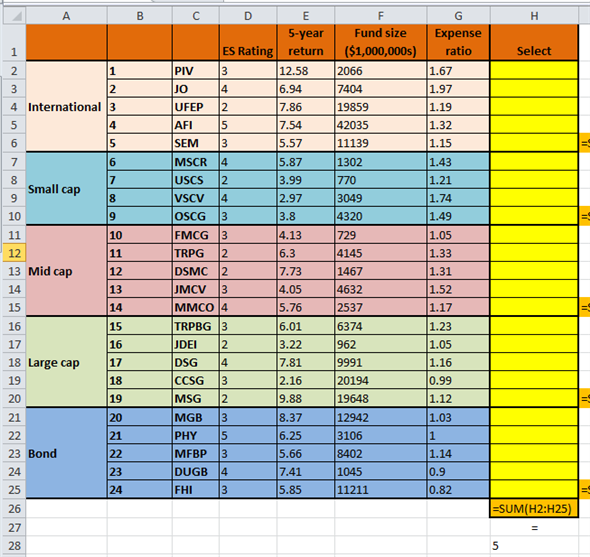Figure 2: Screenshot of formulas used in spreadsheet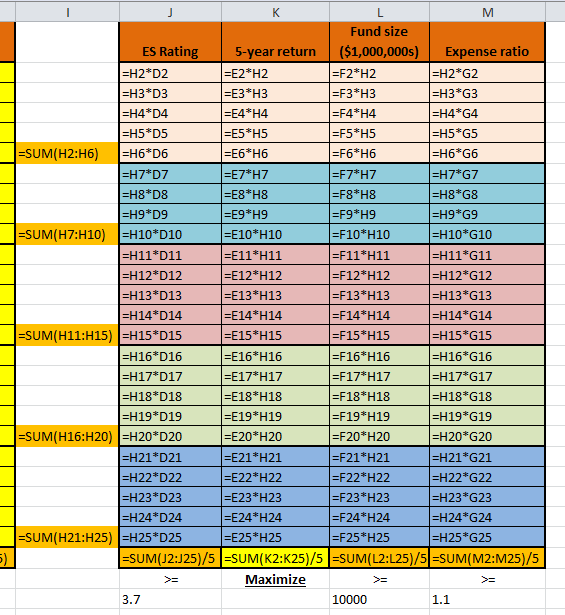Figure 3: Screenshot of formulated spreadsheet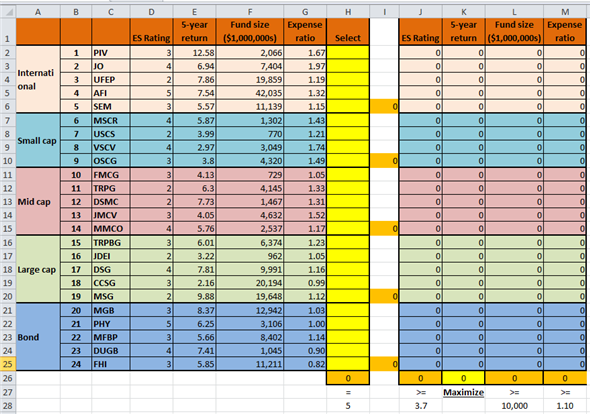Now, click on excel "Solver" option. Put the following values in the "Solver" window: Figure 3: Screenshot of Excel solverClick on "Solve" option. Then, click on "OK" option. It would give the following results: Figure 5: Screenshot of final results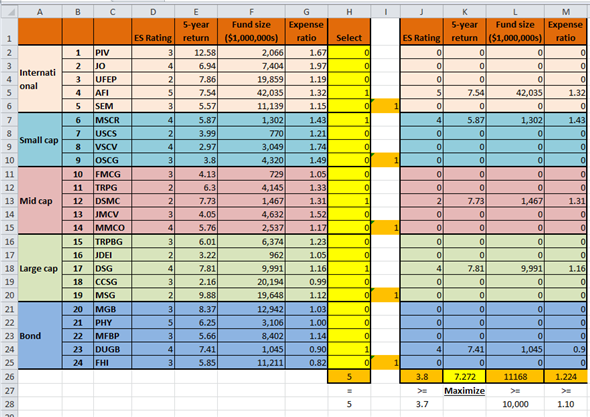Hence, it is concluded that the maximum value of average 5-year returns on the fund would be 7.272. To achieve this objective, Person KT needs to invest in funds 4, 6, 12, 17, and 23. Formulate and solve a linear programming model using excel spreadsheet in order to maximize the ES ratings for Person KT, as shown below: Figure 6: Screenshot of formulas used in spreadsheet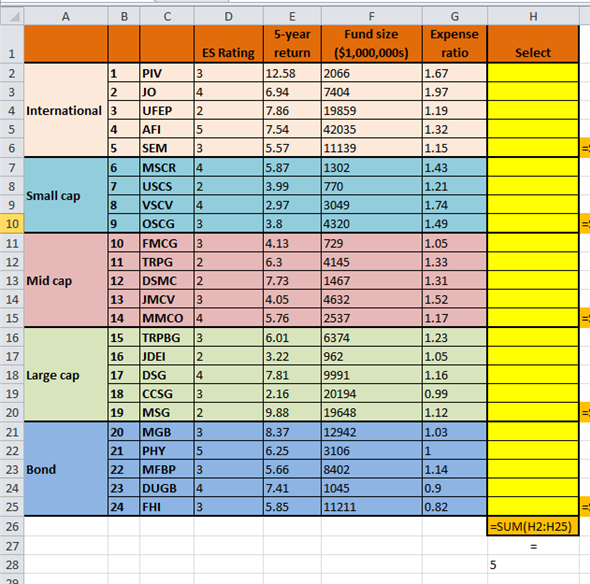Figure 7: Screenshot of formulas used in spreadsheet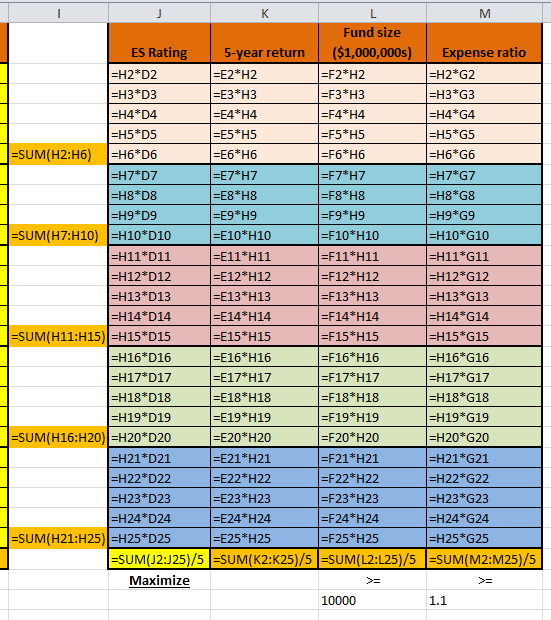Figure 8: Screenshot of formulated spreadsheet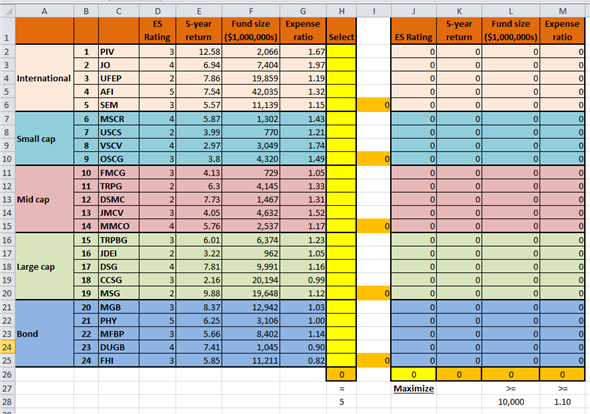Now, click on excel "Solver" option. Put the following values in the "Solver" window: Figure 9: Screenshot of Excel solver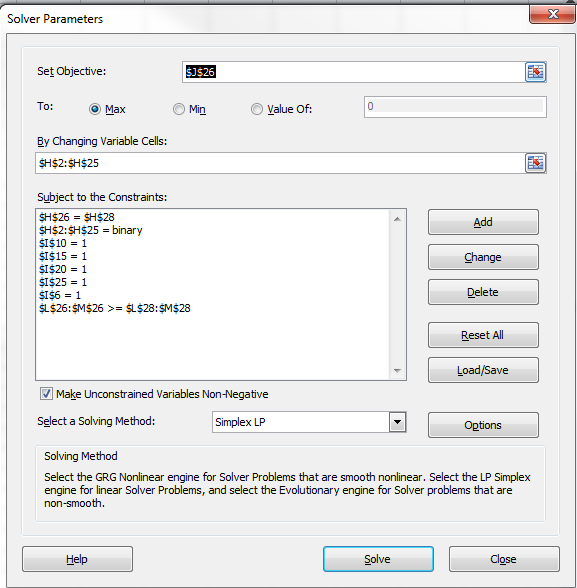Click on "Solve" option. Then, click on "OK" option. It would give the following results: Figure 10: Screenshot of final results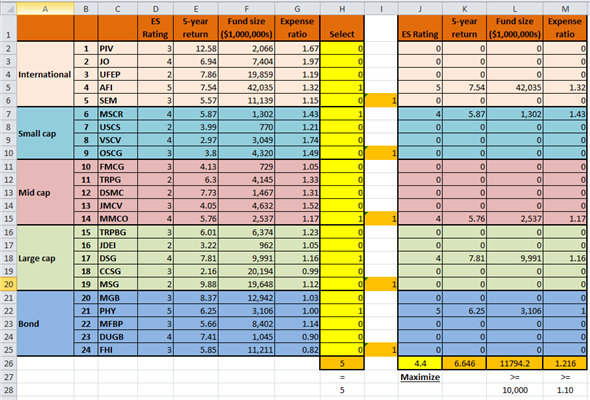Hence, it is concluded that the maximum value of ES rating would be 4.4. To achieve this objective, Person KT needs to invest in funds 4, 6, 14, 19, and 21.

A mathematical optimization technique where all the variables are restricted to be integer is integer linear programing. It is used when fractional solutions are non-realistic. Develop an integer linear programming model by framing the objective function with the given constraints as follows: Define the objective function to maximize the average GPA as shown below: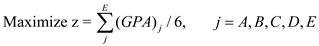Define the constraints as shown below: • Each group must have three members. Therefore,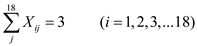• All students should be part of a group. Therefore,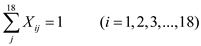• There should be at least one female student in every group. Therefore,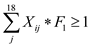• There should be at least one international student in every group. Therefore,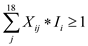• One group must not contain more than two students from same major. Therefore,• The average GPA of each group should at least 2.8. Therefore,Now, formulate MS excel spreadsheet as shown below: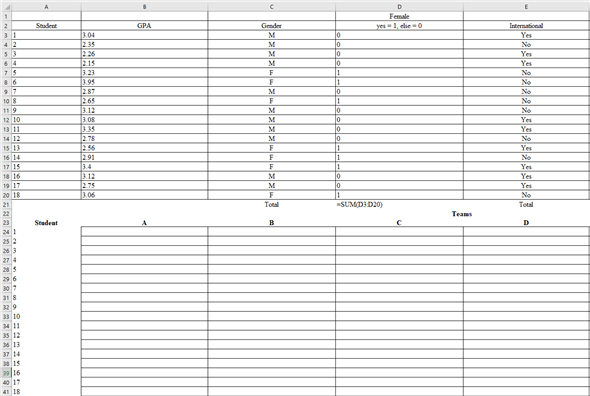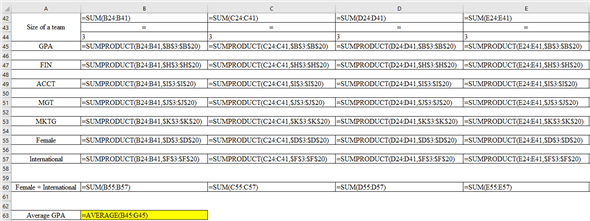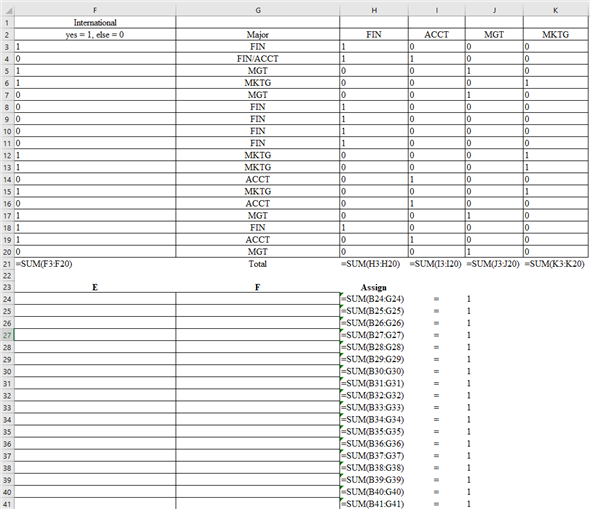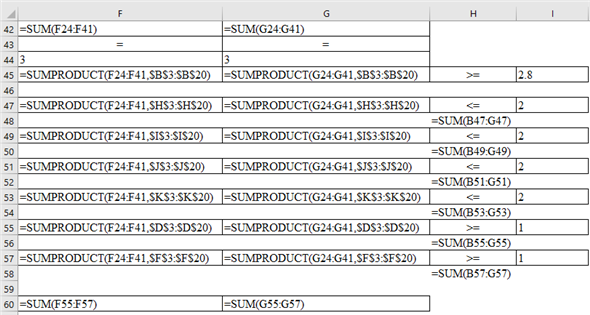The final look of formulated MS excel spreadsheet is provided below: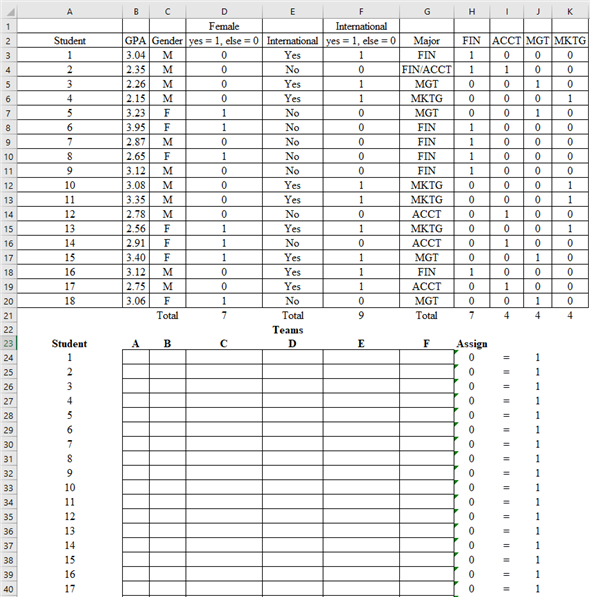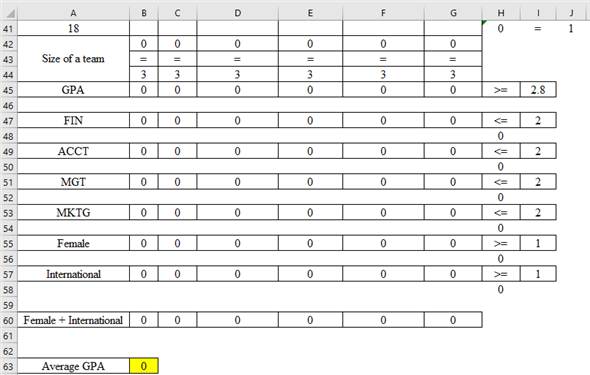Click 'Data', open 'Solver', insert data, and click 'Solve', as shown below: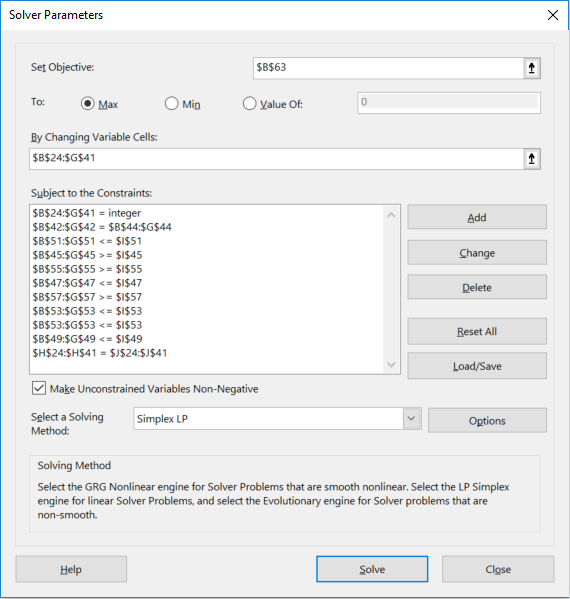The final output is provided below: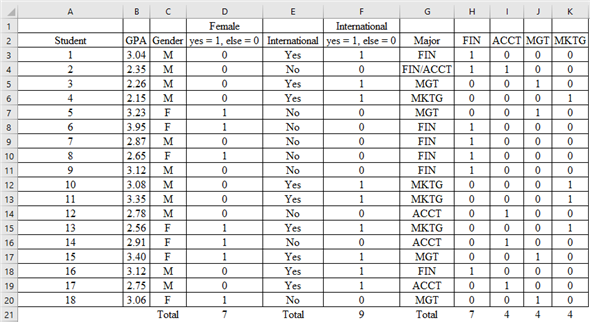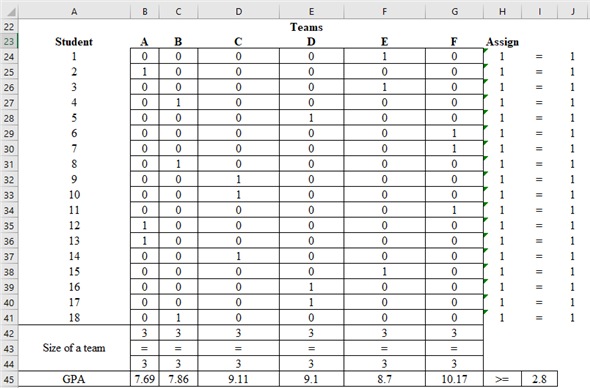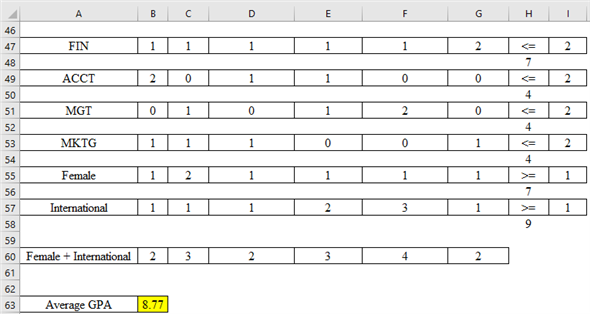Hence, the maximum average GPA of six teams is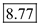and the teams along with members and GPA of each team are provided below: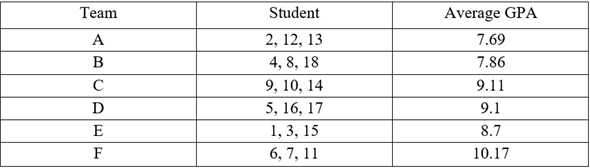As per the calculated GPA of each team, the teams are relatively equal in terms of functionality and academic capability.

Company AMS is a consulting firm that wants to expand its business operations into other locations. The company has four existing offices. It has an option to expand its offices into seven new locations. The budget constraint is \$14,000,000 There are employee constraints for each location both in terms of capacity and demand etc. Assume that the company wants to increase the profit potential. The profit can be maximized through minimization of rank But, if one chooses to minimization of rank the model will constraint locations. To eliminate this complexity let us assign new ranks to the possible locations. No the greatest rank means that the greatest potential for profit: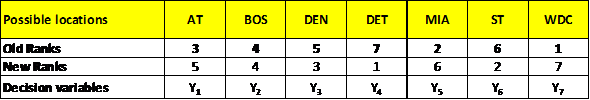Now formulate an integer programming model as shown below: Step-1: Choose decision variables: Let,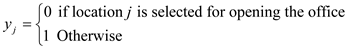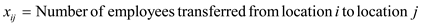Step-2: Formulate the objective function The objective is to maximize the profit potential, write this in mathematical form, as shown below:Step-3: Setup constraints Cost Constraint: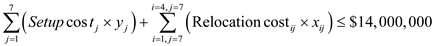Compulsory Location1: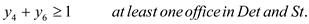Compulsory Location2: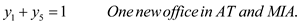Capacity constraintsDemand of selected locations must be fulfilled constraints:Integer constraint: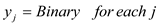Binary constraint: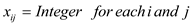Nonnegative constraints :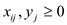Solve the formulated model using excel as shown below: Step-1: Enter data in excel sheet exactly as shown below: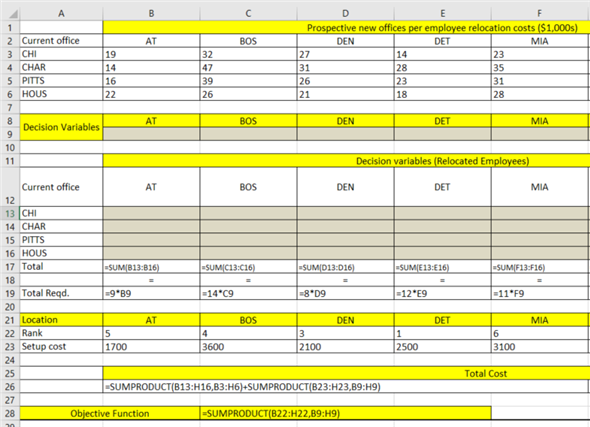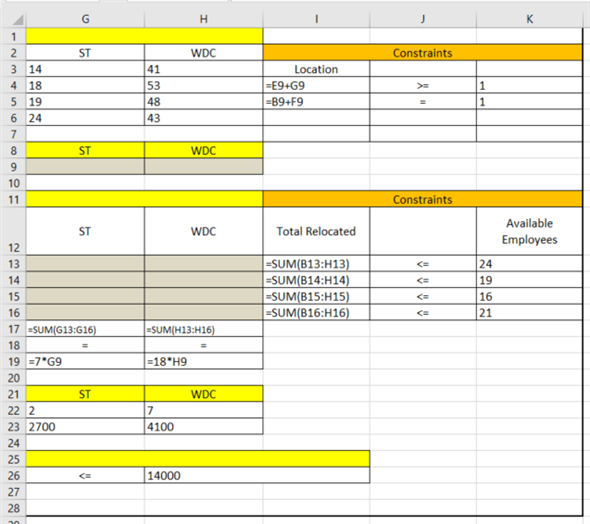Step-2: The model will look like the below model: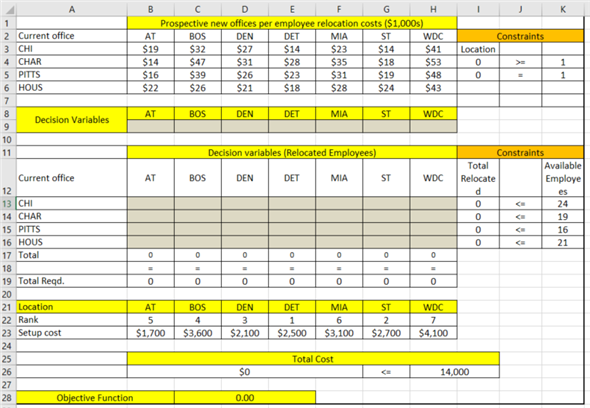Step-3: Open solver: Excel Data-tab Solver, and enter data in the solver as shown below: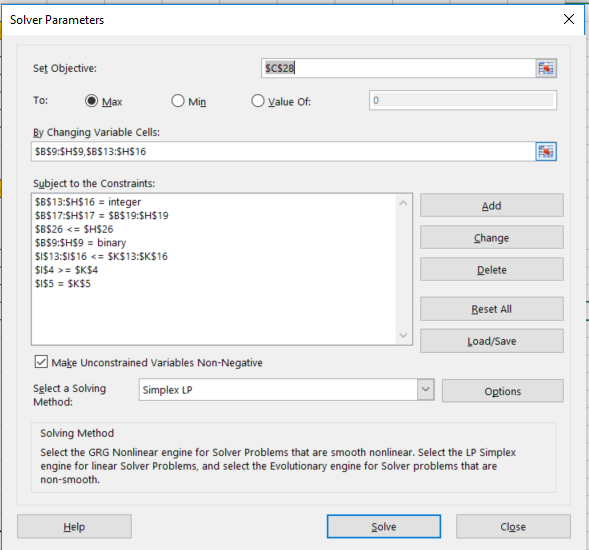Step-4: Clicking on solve will generate the following results: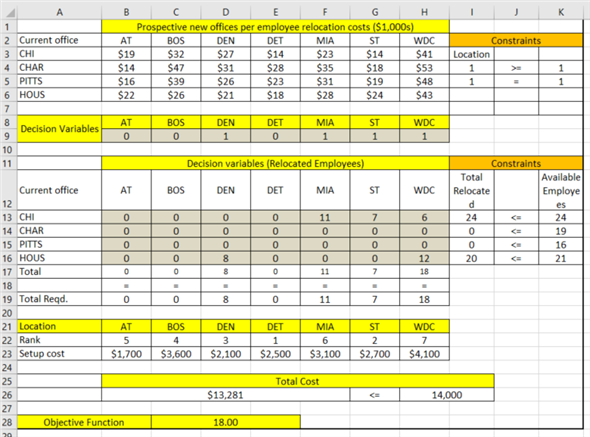Step-5: Interpret the results : Within this budget company the most profit potential decision will be to open offices in location DEN, MIA, ST and WDC. The maximum profit potential in terms of new ranks is 18. Results: 1. The company should open 4 offices. 2. The new offices should be opened in locations DEN, MIA, ST and WDC. 3. The company should transfer 11 employees from CHI to MIA, 7 employees from CHI to ST, and 6 employees from CHI to WDC. Besides, it should transfer 8 employees from HOUS to DEN and 12 employees from HOUS to WDC.

There is no answer for this question

There is no answer for this question

There is no answer for this question

There is no answer for this question

There is no answer for this question

There is no answer for this question